## How to sort numbers within a cell in Excel?

It is easy and common for us to sort numbers in a list of column, but have you ever tried to sort numbers within a single cell? May be there is no good way for you except arrange them one by one, here, I will talk about how to sort numbers within cells in Excel.

Sort numbers within cells with formula

Sort numbers within cells with User Defined Function

Sort numbers which separated by commas within cells with VBA code

####Sort numbers within cells with formula

To sort numbers within cells in a worksheet, you can apply the following long formula, please do as this:

1. Next to your data, please enter the following formula, in this example, I will type it into cell C1, see screenshot:

=TEXT(SUM(SMALL(--MID(A1,ROW(INDIRECT("1:"&LEN(A1))),1),ROW(INDIRECT("1:"&LEN(A1))))*10^(LEN(A1)-ROW(INDIRECT("1:"&LEN(A1))))),REPT("0",LEN(A1)))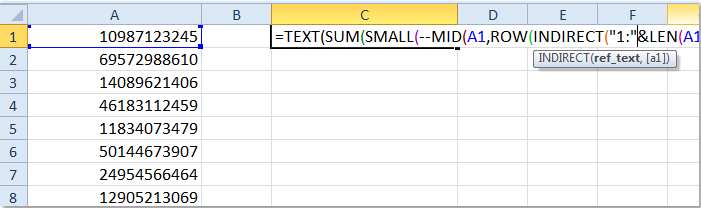2. Then press Ctrl + Shift + Enter keys together, then drag the fill handle over to the range that you want to apply this formula, and you will get the numbers have been sorted from small to large. See screenshot: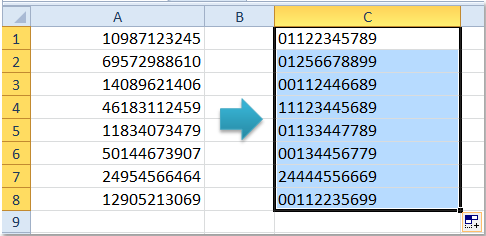Notes:

1. If the digit of the number is more than 15 in the cell, this formula will not get the correct result.

2. If you want to sort the numbers in descending order, you can use this formula: =TEXT(SUM(LARGE(--MID(A1,ROW(INDIRECT("1:"&LEN(A1))),1),ROW(INDIRECT("1:"&LEN(A1))))*10^(LEN(A1)-ROW(INDIRECT("1:"&LEN(A1))))),REPT("0",LEN(A1))).

3. In the above formulas, A1 indicates the cell which contains the numbers you want to sort, you can change it to your need.

####Sort numbers within cells with User Defined Function

As there are some limitations of the formula, you can use the following User Defined Function to sort numbers in cells longer than 15 digits.

1. Hold down the ALT + F11 keys, and it opens the Microsoft Visual Basic for Applications window.

2. Click Insert > Module, and paste the following code in the Module Window.

VBA code: Sort numbers within cells

``````Function SortNumsInCell(pNum As String, Optional pOrder As Boolean) As String
'Update 20140717
Dim xOutput As String
For i = 0 To 9
For j = 1 To UBound(VBA.Split(pNum, i))
xOutput = IIf(pOrder, i & xOutput, xOutput & i)
Next
Next
SortNumsInCell = xOutput
End Function
``````

3. Then save and close this code, go back to your worksheet, and enter this formula =sortnumsincell(A1) into a blank cell next to your data, see screenshot: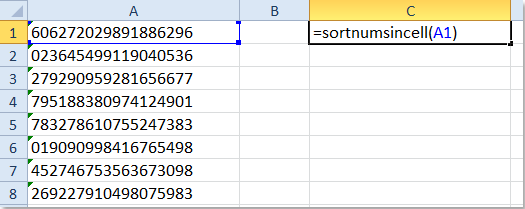4. And then drag the fill handle to the cells that you want to contain this formula, and all the numbers in the cells have been sorted in ascending order as following screenshot shown: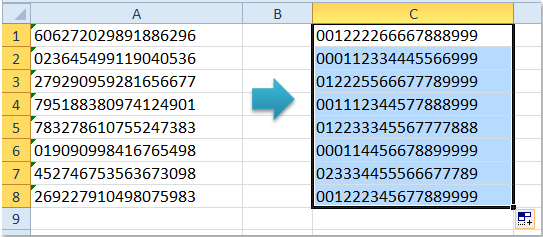Note: If you want to sort the numbers in descending order, please enter this formula =sortnumsincell(A1,1).

####Sort numbers which separated by commas within cells with VBA code

If your numbers are separated by certain characters such as comma, semicolon, period and so on as following screenshot, how could you sort them in cells? Now, I introduce a VBA code for you to sort them.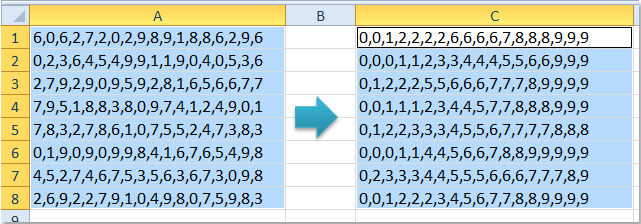1. Hold down the ALT + F11 keys to open the Microsoft Visual Basic for Applications window.

2. Click Insert > Module, and paste the following code in the Module Window.

VBA code: Sort numbers are separated by commas within cells

``````Sub SortNumsInRange()
'Update 20140717
Dim Rng As Range
Dim WorkRng As Range
Dim Arr As Variant
On Error Resume Next
xTitleId = "KutoolsforExcel"
Set WorkRng = Application.Selection
Set WorkRng = Application.InputBox("Range", xTitleId, WorkRng.Address, Type:=8)
Set objArrayList = CreateObject("System.Collections.ArrayList")
For Each Rng In WorkRng
Arr = VBA.Split(Rng.Value, ",")
For i = 0 To UBound(Arr)
xMin = i
For j = i + 1 To UBound(Arr)
If Arr(xMin) > Arr(j) Then
xMin = j
End If
Next j
If xMin <> i Then
temp = Arr(i)
Arr(i) = Arr(xMin)
Arr(xMin) = temp
End If
Next i
Rng.Value = VBA.Join(Arr, ",")
Next
End Sub
``````

3. Then press F5 key to run this code, and then select your cells which contain the numbers in the popped out prompt box, see screenshot: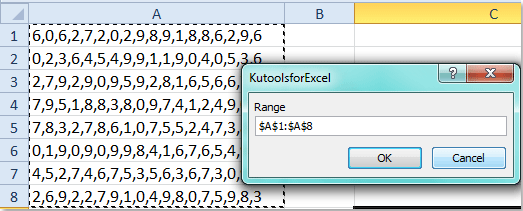4. And then click OK, all the numbers in the cells have been sorted ascendingly in the original range.

Note: You can change the comma “,” to any other characters as you need in the above code. And this code only can sort data ascendingly.

Related articles:

How to sort numbers with hyphens in Excel?

How to sort email address by domain in Excel?

How to sort rows to put the blank cells on top in Excel?

### Best Office Productivity Tools

 Popular Features: Find, Highlight or Identify Duplicates   |  Delete Blank Rows   |  Combine Columns or Cells without Losing Data   |   Round without Formula ... Super Lookup: Multiple Criteria VLookup  |   Multiple Value VLookup  |   VLookup Across Multiple Sheets   |   Fuzzy Lookup .... Advanced Drop-down List: Quickly Create Drop Down List   |  Dependent Drop Down List   |  Multi-select Drop Down List .... Column Manager: Add a Specific Number of Columns  |  Move Columns  |  Toggle Visibility Status of Hidden Columns  |  Compare Ranges & Columns ... Featured Features: Grid Focus   |  Design View   |   Big Formula Bar   |  Workbook & Sheet Manager   |  Resource Library (Auto Text)   |  Date Picker   |  Combine Worksheets   |  Encrypt/Decrypt Cells   |  Send Emails by List   |  Super Filter   |   Special Filter (filter bold/italic/strikethrough...) ... Top 15 Toolsets:  12 Text Tools (Add Text, Remove Characters, ...)   |   50+ Chart Types (Gantt Chart, ...)   |   40+ Practical Formulas (Calculate age based on birthday, ...)   |   19 Insertion Tools (Insert QR Code, Insert Picture from Path, ...)   |   12 Conversion Tools (Numbers to Words, Currency Conversion, ...)   |   7 Merge & Split Tools (Advanced Combine Rows, Split Cells, ...)   |   ... and more

Supercharge Your Excel Skills with Kutools for Excel, and Experience Efficiency Like Never Before. Kutools for Excel Offers Over 300 Advanced Features to Boost Productivity and Save Time.  Click Here to Get The Feature You Need The Most...#### Office Tab Brings Tabbed interface to Office, and Make Your Work Much Easier

• Enable tabbed editing and reading in Word, Excel, PowerPoint, Publisher, Access, Visio and Project.
• Open and create multiple documents in new tabs of the same window, rather than in new windows.
• Increases your productivity by 50%, and reduces hundreds of mouse clicks for you every day!No ratings yet. Be the first to rate!
This comment was minimized by the moderator on the site
Hola, se pueden ordenar letras en una sola celda por orden alfabético? Yo uso excel para Mac. Gracias
This comment was minimized by the moderator on the site
Hi thanks for nice good for sorting numbers which separated by commas within cells with VBA code
Just I have faced one problem with the code.
The code cannot detect three digit number. for example the numbers (65, 93, 53, 72, 64, 85, 103, 48, 77, 81, 54) after applying the code, the new order (103, 48, 53, 54, 64, 65, 72, 77, 81, 85, 93)
Do you have any solution for the problem?
This comment was minimized by the moderator on the site
<div data-tag="code">Public Function CellSort(r As Range) As String
Dim bry() As Long, L As Long, U As Long
ch = r(1).Text
ary = Split(ch, ",")
L = LBound(ary)
U = UBound(ary)
ReDim bry(L To U)
For i = LBound(ary) To UBound(ary)
bry(i) = CLng(ary(i))
Next i
Call BubbleSort(bry)
For i = LBound(bry) To UBound(bry)
ary(i) = CStr(bry(i))
Next i
CellSort = Join(ary, ",")
End Function

Sub BubbleSort(arr)
Dim strTemp As Variant
Dim i As Long
Dim j As Long
Dim lngMin As Long
Dim lngMax As Long
lngMin = LBound(arr)
lngMax = UBound(arr)
For i = lngMin To lngMax - 1
For j = i + 1 To lngMax
If arr(i) > arr(j) Then
strTemp = arr(i)
arr(i) = arr(j)
arr(j) = strTemp
End If
Next j
Next i
End SubAfter inserting the above code, please apply this formula: =CellSort(A1).And you will get the result you need.
This comment was minimized by the moderator on the site
How to sort A-Z text within a cell in Excel?
This comment was minimized by the moderator on the site
hOLA, MI PROBLEMA ES QUE TENGO EXEL 2019 EN ESPAÑOL COMO SERIA LA FORMULA?
This comment was minimized by the moderator on the site
Hi, the VBA code seems to output incorrectly, example before 13,50,47,7,39 and after 13-39-47-50-7. Any ideas why?
This comment was minimized by the moderator on the site
i want to sort total an amount 14000 to 20000 from various row Example:- 2000,1500 one row and like that all row amount to arrange
This comment was minimized by the moderator on the site
need to sort 84-12-74-26-98 any order 12-26-74-84-98 or 98-84-74-26-12 thank you
This comment was minimized by the moderator on the site
If CInt(Arr(xMin)) > CInt(Arr(j)) and it works
This comment was minimized by the moderator on the site
Is there is any way to sort more numbers in same time from one cell? Example, i have a list of 50000 asset numbers such as A1234,A1235... and i need to pull 500 specific numbers and i need to pull 500 at the time to make change and save.Thank you
This comment was minimized by the moderator on the site
I have a series of cells with numbers separated by a space that I want to sort. eg 8 4 5 1 6 3 that I want to sort as 1 3 4 5 6 8 Any help appreciated
This comment was minimized by the moderator on the site
Hi, I was wondering how this UDF, =sortnumsincell(A1,1), can be modified more generally, like =sortnumsincell(A1," "," ",,1) where the first argument, A1, is the target cell, the second argument," ", is a delimiter that could take any character, or a space, or nothing, with third argument, " ", a different or same delimiter, and the fourth argument, 1 or 0, indicating an ascending or descending sort, with the result string displaying, correctly sorted, within one cell, with delimiter default same as the original string unless specified in the third term. I would like it to work both on string and numerical, and sometimes the second or third argument might be a line feed, as would be manually entered with alt-enter. You'd be my hero of the month if you could do that. I tried but failed miserably. Thank you.
There are no comments posted here yet